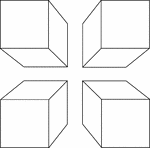4 Congruent Rectangular Prisms

Illustration of 4 congruent rectangular prisms placed in the shape of a square. They are arranged to…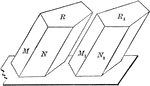Two Equal Prisms

Diagram used to prove the theorem: "Two prisms are equal when the three faces about a trihedral of one…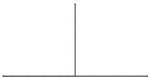Supplementary Angles 90 - 90

Supplementary Angles degrees 90 and 90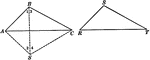Equal Triangles

Illustration used to show that two triangles are equal if the three sides of one are equal respectively…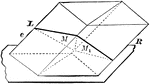Parallelopiped Divided Into Triangular Prisms

Diagram used to prove the theorem: "The plane passed through two diagonally opposite edges of a parallelopiped…